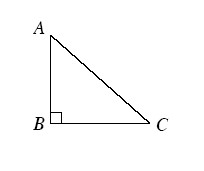### Home > CCG > Chapter 10 > Lesson 10.2.3 > Problem10-106

10-106.

For the triangle at right, find each trigonometric ratio below. The first one is done for you.

Refer to the Math Notes box in Lesson 5.1.2 if you need help remembering any trigonometric ratios.

1. $\tan C=\frac{AB}{BC}$

1. $\sin C$

1. $\tan A$

1. $\cos C$

$\frac{BC}{AC}$

1. $\cos A$

1. $\sin A$

$\frac{BC}{AC}$